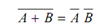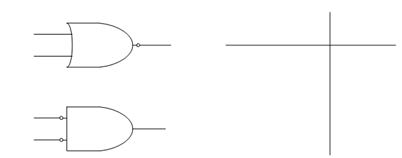## De Morgans Theorems Assignment Help

Assignment Help: >> Logic Gates and Boolean algebra - De Morgans Theorems

De Morgan's Theorems:

De Morgan's First Theorem

It says that complement of sum equals the product of complements.The LHS of the equation explain a NOR (NOT-OR) gate and the RHS of the equation explain an AND gate with inverted inputs and both of the equations have the same truth table as illustrated in FigureFigure: (a) NOR Logic Gate, (b) AND Gate with Inverted Inputs, and (c) Truth Tble